orRegisteror

## How to sum all digits in a number in Excel?

If you have a cell which contains a value, and now, you want to add all the digits together of the cell. For example, if you have the value 12345 in a cell, you want to do this calculation: 1+2+3+4+5, and get the value 15. Are there any good ways for you to sum all digits of a number in Excel?

Sum all digits in a numbers with formulas

Sum all digits in a numbers with User Defined Function

 Sum all individual numbers within a cell: With Kutools for Excel’s Sum numbers in a cell formula, you can quickly sum all digits of a number together in a cell.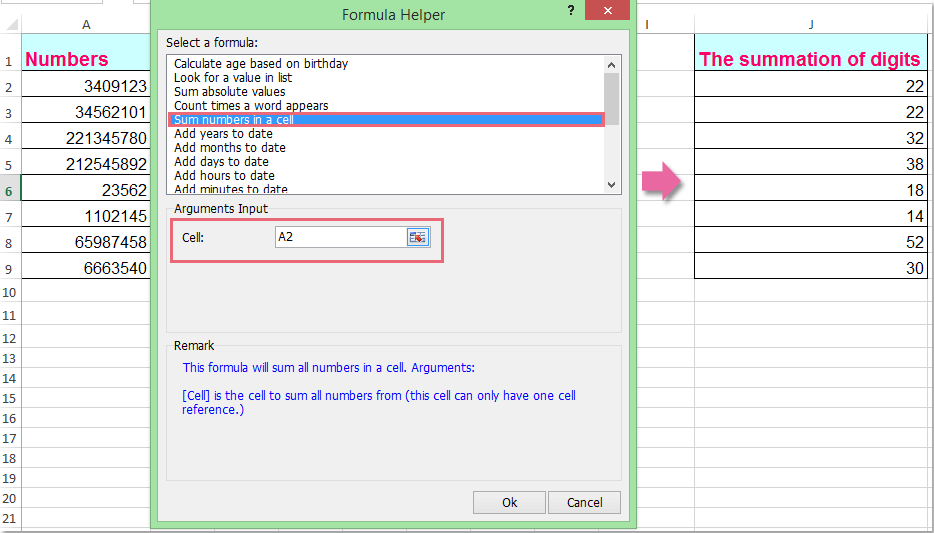Kutools for Excel: with more than 200 handy Excel add-ins, free to try with no limitation in 60 days.###### Save 50% of your time, and reduce thousands of mouse clicks for you every day!

Without adding the digits one by one manually, the following formulas can help you get the summation of a cell quickly.

1. Please enter this formula =SUMPRODUCT(1*MID(A2,ROW(INDIRECT("1:"&LEN(A2))),1))(A2 contains the number which you want to sum its digits, you can change it to your need) into a blank cell beside your data, in this example, I will enter it in cell B2. See screenshot: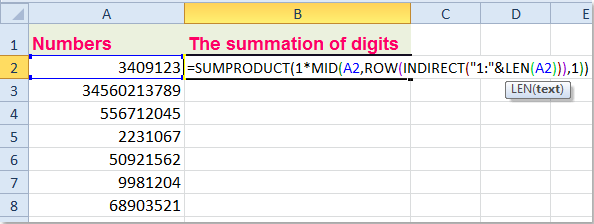2. Then press Enter key to return the result, and select the cell B2, then drag the fill handle over to the range that you want to apply this formula, and you will get the summation of digits of each cell number.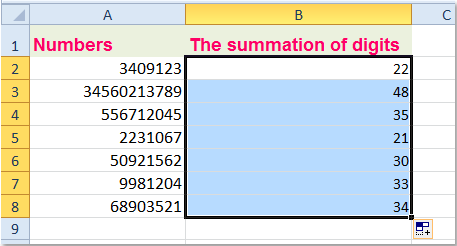Notes:

1. Except the above formula, you can also use an array formula: =SUM(1*MID(A2,ROW(INDIRECT("1:"&LEN(A2))),1)), and remember to press Ctrl + Shift + Enter keys together to get the correct result.

2. These formulas work fine if the number is a positive whole number. If there is negative sign or a decimal point in the number, the formulas return a #VALUE! error.

To add up all digits of a cell number, the following VBA code also can help you.

1. Hold down the ALT + F11 keys, and it opens the Microsoft Visual Basic for Applications window.

2. Click Insert > Module, and paste the following code in the Module Window.

VBA code: Sum all digits of a cell number

``` Function SumDigits(ByVal Number As Long) As Long
'Update 20140905
Do While Number >= 1
SumDigits = SumDigits + Number Mod 10
Number = Int(Number / 10)
Loop
End Function
```

3. Then save and close this code, return to the worksheet, and enter this formula =SumDigits(A2) into a blank cell, see screenshot: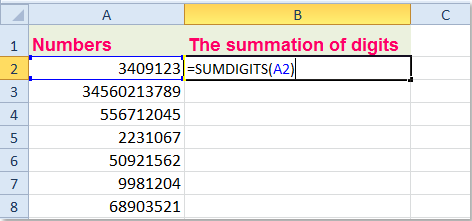4. Then drag the fill handle down to the cells that you want to contain this formula, you will get the following result.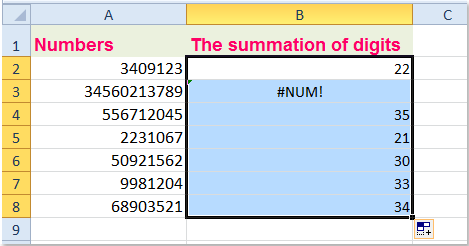Notes:

1. If the digit of your number longer or equal 11, you will get an error value.

2. If the number contain negative sign, the result will be zero; if the number contain decimal point, the result will be wrong.

Here, I can also talk about a useful tool- Kutools for Excel, with its Sum numbers in a cell function, you can quickly add up all digits within a number.

 : with more than 300 handy Excel add-ins, free to try with no limitation in 60 days.

After installing Kutools for Excel, please do as follows:

1. Click a blank cell where you want to output the result.

2. Then click Kutools > Formulas > Sum numbers in a cell, see screenshot: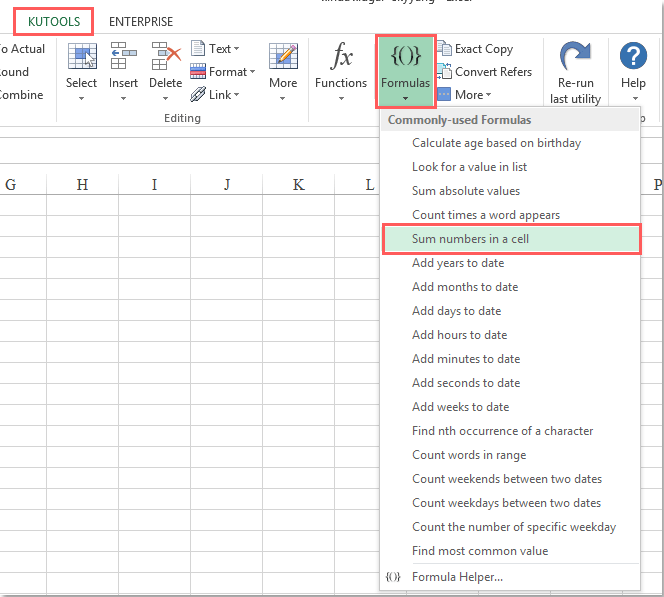3. In the Formula Helper dialog box, click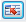button to select the cell value that you want to sum the digits, see screenshot: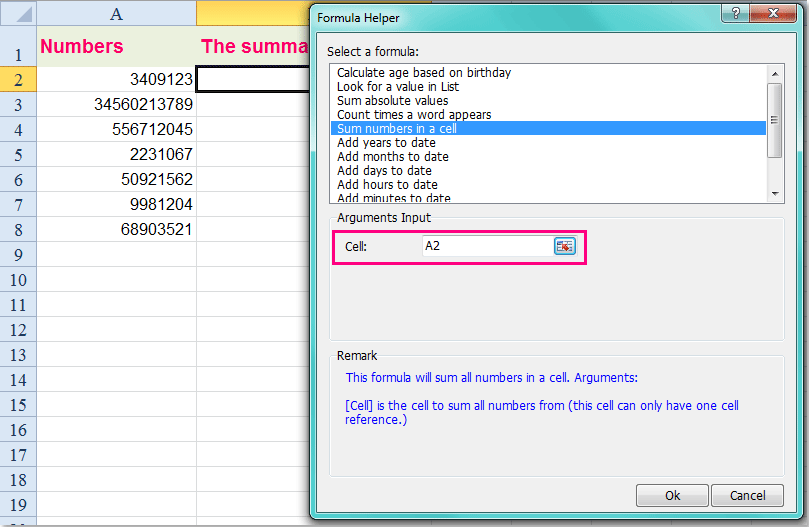4. Then click Ok button, and drag the fill handle over to the cells that you want to sum the digits in the cells. And all the digits in each cell have been added together, see screenshot: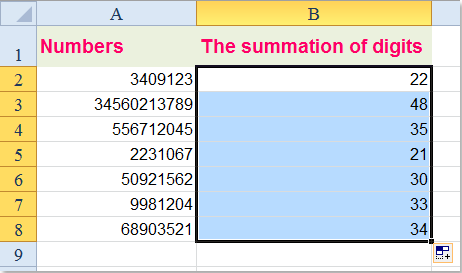Kutools for Excel: with more than 200 handy Excel add-ins, free to try with no limitation in 60 days.

Related article:

How to sum diagonal of a range in Excel?

### The Best Office Productivity Tools

#### Kutools for Excel Solves Most of Your Problems, and Increases Your Productivity by 80%

• Reuse: Quickly insert complex formulas, charts and anything that you have used before; Encrypt Cells with password; Create Mailing List and send emails...
• Super Formula Bar (easily edit multiple lines of text and formula); Reading Layout (easily read and edit large numbers of cells); Paste to Filtered Range...
• Merge Cells/Rows/Columns without losing Data; Split Cells Content; Combine Duplicate Rows/Columns... Prevent Duplicate Cells; Compare Ranges...
• Select Duplicate or Unique Rows; Select Blank Rows (all cells are empty); Super Find and Fuzzy Find in Many Workbooks; Random Select...
• Exact Copy Multiple Cells without changing formula reference; Auto Create References to Multiple Sheets; Insert Bullets, Check Boxes and more...
• Extract Text, Add Text, Remove by Position, Remove Space; Create and Print Paging Subtotals; Convert Between Cells Content and Comments...
• Super Filter (save and apply filter schemes to other sheets); Advanced Sort by month/week/day, frequency and more; Special Filter by bold, italic...
• Combine Workbooks and WorkSheets; Merge Tables based on key columns; Split Data into Multiple Sheets; Batch Convert xls, xlsx and PDF...
• More than 300 powerful features. Supports Office/Excel 2007-2019 and 365. Supports all languages. Easy deploying in your enterprise or organization. Full features 30-day free trial. 60-day money back guarantee.#### Office Tab Brings Tabbed interface to Office, and Make Your Work Much Easier

• Enable tabbed editing and reading in Word, Excel, PowerPoint, Publisher, Access, Visio and Project.
• Open and create multiple documents in new tabs of the same window, rather than in new windows.
• Increases your productivity by 50%, and reduces hundreds of mouse clicks for you every day!Say something here...
symbols left.
###### or post as a guest, but your post won't be published automatically.
• To post as a guest, your comment is unpublished.
· 1 months ago
I typed 3 zero in 2nd column and put sum formula i.e. 50 in 1st column and three zero in 2nd column but excel is not working because the zero are going to remove automatically. Could you please help me.
• To post as a guest, your comment is unpublished.
· 1 months ago
Hello, Murtuza,
Thank you!
• To post as a guest, your comment is unpublished.
· 4 months ago
How to count number in a cell in excel i.e. for example : 1+1+2+3+4+5+6+7 = 8
• To post as a guest, your comment is unpublished.
· 5 months ago
Thank you so much
• To post as a guest, your comment is unpublished.
· 8 months ago
I am trying to find the avg of multipule numbers in a cell, lets say 22,11,18,14,3,6 are all in the same cell is there a way for another cell to display 12.3?
• To post as a guest, your comment is unpublished.
· 8 months ago
Hello, john,
To calculate the average of multiple numbers within a cell, please apply the below formula:
=IF(A1="","",ROUND(SUMPRODUCT(--(0&TRIM(MID(SUBSTITUTE(A1,",",REPT(" ",99)),ROW(\$1:\$99)*99-98,99))))/(LEN(A1)-LEN(SUBSTITUTE(A1,",",))+1),2))
Note: the last number 2 in the formula indicates that retain two decimal places after rounding. You can change it to your need.

• To post as a guest, your comment is unpublished.
· 1 years ago
Before i ask i apologize about some rude and hard -to-understand expressions which could be in follow sentences caused by my poor English skill in advance.

I could use this functions and solve the problem though, I actually couldn't get how the function work.

This is the function :
=SUM(1*MID(A2,ROW(INDIRECT("1:"&LEN(A2))),1))

I know functions "SUM", "MID", "ROW", and "INDIRECT", but I have no idea about expressions "1*MID" and ""1:"&" at all.

Can someone give me any answer or clue which can make me understand?
• To post as a guest, your comment is unpublished.
· 1 years ago
Hi,
but I need to have a one number at the end, but your formula is giving two numbers.
can you help?
regards
• To post as a guest, your comment is unpublished.
· 1 years ago
Great code!
How to repeat the process into 1 digit with VBA code?
Example: 463 > 4+6+3=13 > 1+3=4
• To post as a guest, your comment is unpublished.
· 1 years ago
In same way how do i multiply
i have some excel numbers like Exm : 445, 556 , 713 . ( 4*4*5 ) i want multiplication of this
thnx
• To post as a guest, your comment is unpublished.
· 1 years ago
Hi, Shriyan,
To multiply all numbers within a cell, you can apply the following user defined function:

Function MultiplyDigits(ByVal Number As Long) As Long
'Update 20140905
MultiplyDigits = 1
Do While Number >= 1
MultiplyDigits = MultiplyDigits * (Number Mod 10)
Number = Int(Number / 10)
Loop
End Function

And then apply this formula: =MultiplyDigits(A2)

• To post as a guest, your comment is unpublished.
· 1 years ago
oh thank you so much for u r reply Skyyang
• To post as a guest, your comment is unpublished.
· 1 years ago
This is not all possible permutations. If I put 123, how can I get the 27 permutations. That means, including 111 etc.
• To post as a guest, your comment is unpublished.
· 1 years ago
How can I add the values in a cell. For example if i have a huge number like 4583789484858 in a cell and I want them added together in such a way that
the system does 4+5+8+3+7+8+9+4+8+4+8+5+8 to give me result of 81?
• To post as a guest, your comment is unpublished.
· 4 years ago

thanks
• To post as a guest, your comment is unpublished.
· 5 years ago
I'm trying to figure out a formula using arrays to add/subtract a number from each digit in a cell. Example: If A1 contains 7721, then I'd like B1 to contain 2278 (subtracting 9 from each digit in A1). I have tried and tried to figure this out without success. Thank you!
• To post as a guest, your comment is unpublished.
· 1 years ago
if i have decimal number for example 22.2456 in excel how can i Sum for All this Digits ?
• To post as a guest, your comment is unpublished.
· 1 years ago
Hi, Rehan,
To sum all digits in decimal number, the following code may help you.

Function SumDigits(Number As String) As Integer
Dim x As Integer, C As String
For x = 1 To Len(Number)
C = Mid(Number, x, 1)
If IsNumeric(C) Then SumDigits = SumDigits + C
Next
End Function

And then apply this formula: =SumDigits(A1).